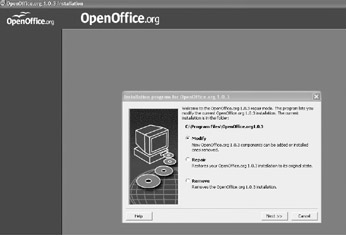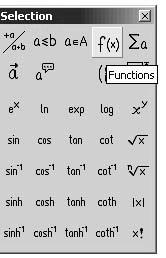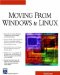# Math

 < Day Day Up >

If you have ever needed to create a document that contained mathematical equations, then you know it is not easy to find an application that combines the required formatting and printing of word processing and the representation of mathematical equations. Most word processors have very limited capability in this regard. Even Microsoft Word and its companion Math Equation Editor do not fully accomplish this goal. This is another area in which Open Office has a superior performance. Open Office Math is essentially a mathematical word processor. You can use it to create documents that contain mathematical equations. This is very useful for mathematicians, engineers, astronomers, chemists, physicists, or students taking courses in any of these disciplines. You can see the basic Open Office Math screen shown in Figure 11.27.Figure 11.27: Open Office Math.

Notice the small box in the upper-right corner with various mathematical symbols in it. In this box you can select various types of symbols. The top row contains the types. As you pass your mouse over them, as shown in Figure 11.28, a tool will show you what type it is. If you click on that type, you will see a different set of symbols in the main window.Figure 11.28: The symbol types.

You can then use a combination of those symbols and your own typing to create mathematical formula documents that you can save, edit, open, or print just like any standard text document. So with Open Office Math, you have a word processor for mathematics.

 < Day Day Up >Moving From Windows To Linux (Charles River Media Networking/Security)
ISBN: 1584502800
EAN: 2147483647
Year: 2004
Pages: 247
Authors: Chuck Easttom